# Fraction calculator

The calculator performs basic and advanced operations with fractions, expressions with fractions combined with integers, decimals, and mixed numbers. It also shows detailed step-by-step information about the fraction calculation procedure. Solve problems with two, three, or more fractions and numbers in one expression.

## Result:

### 41/7 + 11/2 = 79/14 = 5 9/14 ≅ 5.6428571

Spelled result in words is seventy-nine fourteenths (or five and nine fourteenths).

### How do you solve fractions step by step?

1. Conversion a mixed number 4 1/7 to a improper fraction: 4 1/7 = 4 1/7 = 4 · 7 + 1/7 = 28 + 1/7 = 29/7

To find a new numerator:
a) Multiply the whole number 4 by the denominator 7. Whole number 4 equally 4 * 7/7 = 28/7
b) Add the answer from previous step 28 to the numerator 1. New numerator is 28 + 1 = 29
c) Write a previous answer (new numerator 29) over the denominator 7.

Four and one seventh is twenty-nine sevenths
2. Conversion a mixed number 1 1/2 to a improper fraction: 1 1/2 = 1 1/2 = 1 · 2 + 1/2 = 2 + 1/2 = 3/2

To find a new numerator:
a) Multiply the whole number 1 by the denominator 2. Whole number 1 equally 1 * 2/2 = 2/2
b) Add the answer from previous step 2 to the numerator 1. New numerator is 2 + 1 = 3
c) Write a previous answer (new numerator 3) over the denominator 2.

One and one half is three halfs
3. Add: 29/7 + 3/2 = 29 · 2/7 · 2 + 3 · 7/2 · 7 = 58/14 + 21/14 = 58 + 21/14 = 79/14
For adding, subtracting, and comparing fractions, it is suitable to adjust both fractions to a common (equal, identical) denominator. The common denominator you can calculate as the least common multiple of both denominators - LCM(7, 2) = 14. In practice, it is enough to find the common denominator (not necessarily the lowest) by multiplying the denominators: 7 × 2 = 14. In the following intermediate step, it cannot further simplify the fraction result by canceling.
In other words - twenty-nine sevenths plus three halfs = seventy-nine fourteenths.

#### Rules for expressions with fractions:

Fractions - simply use a forward slash between the numerator and denominator, i.e., for five-hundredths, enter 5/100. If you are using mixed numbers, be sure to leave a single space between the whole and fraction part.
The slash separates the numerator (number above a fraction line) and denominator (number below).

Mixed numerals (mixed fractions or mixed numbers) write as integer separated by one space and fraction i.e., 1 2/3 (having the same sign). An example of a negative mixed fraction: -5 1/2.
Because slash is both signs for fraction line and division, we recommended use colon (:) as the operator of division fractions i.e., 1/2 : 3.

Decimals (decimal numbers) enter with a decimal point . and they are automatically converted to fractions - i.e. 1.45.

The colon : and slash / is the symbol of division. Can be used to divide mixed numbers 1 2/3 : 4 3/8 or can be used for write complex fractions i.e. 1/2 : 1/3.
An asterisk * or × is the symbol for multiplication.
Plus + is addition, minus sign - is subtraction and ()[] is mathematical parentheses.
The exponentiation/power symbol is ^ - for example: (7/8-4/5)^2 = (7/8-4/5)2

#### Examples:

subtracting fractions: 2/3 - 1/2
multiplying fractions: 7/8 * 3/9
dividing Fractions: 1/2 : 3/4
exponentiation of fraction: 3/5^3
fractional exponents: 16 ^ 1/2
adding fractions and mixed numbers: 8/5 + 6 2/7
dividing integer and fraction: 5 ÷ 1/2
complex fractions: 5/8 : 2 2/3
decimal to fraction: 0.625
Fraction to Decimal: 1/4
Fraction to Percent: 1/8 %
comparing fractions: 1/4 2/3
multiplying a fraction by a whole number: 6 * 3/4
square root of a fraction: sqrt(1/16)
reducing or simplifying the fraction (simplification) - dividing the numerator and denominator of a fraction by the same non-zero number - equivalent fraction: 4/22
expression with brackets: 1/3 * (1/2 - 3 3/8)
compound fraction: 3/4 of 5/7
fractions multiple: 2/3 of 3/5
divide to find the quotient: 3/5 ÷ 2/3

The calculator follows well-known rules for order of operations. The most common mnemonics for remembering this order of operations are:
PEMDAS - Parentheses, Exponents, Multiplication, Division, Addition, Subtraction.
BEDMAS - Brackets, Exponents, Division, Multiplication, Addition, Subtraction
BODMAS - Brackets, Of or Order, Division, Multiplication, Addition, Subtraction.
GEMDAS - Grouping Symbols - brackets (){}, Exponents, Multiplication, Division, Addition, Subtraction.
Be careful, always do multiplication and division before addition and subtraction. Some operators (+ and -) and (* and /) has the same priority and then must evaluate from left to right.

## Fractions in word problems:

• ExpressionsLet k represent an unknown number, express the following expressions: 1. The sum of the number n and two 2. The quotient of the numbers n and nine 3. Twice the number n 4. The difference between nine and the number n 5. Nine less than the number n
• 1700 plans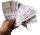1700 plans to budget, 2/5 for rent and 1/6 electricity. What on these two items together?
• Of the 2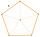Of the shapes,1/6 are triangles, and 5/12 are pentagons. What fraction of the shapes are either triangles or pentagons?
• Math homeworkIt took Jose two-thirds of an hour to complete his math homework on Monday, three-fourths of an hour on Tuesday, any two-fifths of an hour on Wednesday. How many hours did it take Jose to complete his homework altogether?
• EvaluateThe division of numbers 6 and 3 increase by-product of the numbers 115 and 0.1
• A shopkeeper 3A shopkeeper sells 8 1/3 kg, 10 1/4 kg and 11 1/5 kg of apples on 3 consecutive days. What is the total weight of apples sold?
• Mixing colours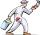Last summer, Mang Tinoy repainted his car. He mixed 2 3/8 cans of white paint, 1 1/3 cans of red paint, and 1 2/4 cans of blue paint. How much paint did he used overall?
• Math: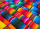Math: solve each problem. 1. for the school's intramurals, a group of students prepared 23 1/2 liters of lemonade to sell. They had 3 5/8 liters leftover. How many liters of lemonade were sold? 2. My mother bought 3 3/4 kg of beef, 23/5 kg of pork, and 5
• Bathroom 4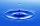Dolor puts 3 1/2 pails of water into a water container in the bathroom every day. Her daughter, Lei, uses 2 1/4 pails of water every day when taking a bath. If the water container had 5 5/8 pails of water at the start, how much water is left in it after 5
• The Mayflower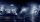The Mayflower traveled for 66 days on the trip from England to America. The weather was storming for many days of their trip. If one and a half of the days at Sea where Sunny with good weather, 1/6 of the days were sunny but very windy and the other days
• One quarter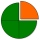Which of the following has a sum of 3/4? A. 1/2+1/4 B. 1/2+1/3 C. 1/4+1/8 D. 1/9+1/12​
• Berry SmoothieRory has 5/8 cup of milk. How much milk does she have left after she doubles the recipe of the smoothie? Berry Smoothie: 2 cups strawberries 1 cup blueberries 1/4 cup milk 1 tbsp (tablespoon) sugar 1/2 tsp (teaspoon) lemon juice 1/8 tsp (teaspoon) vanilla
• Pizza fractionsAnn ate a third of a pizza and then another quater. Total part of pizza eaten by Ann and how much pizza is left?